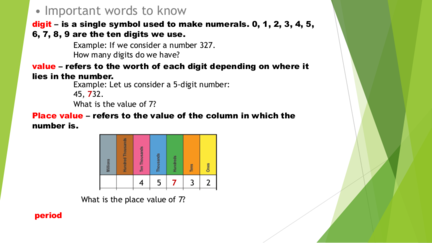# Important Words to knowContributed by:Important words to know
1. • Important words to know
digit – is a single symbol used to make numerals. 0, 1, 2, 3, 4, 5,
6, 7, 8, 9 are the ten digits we use.
Example: If we consider a number 327.
How many digits do we have?
value – refers to the worth of each digit depending on where it
lies in the number.
Example: Let us consider a 5-digit number:
45, 732.
What is the value of 7?
Place value – refers to the value of the column in which the
number is.
4 5 7 3 2
What is the place value of 7?